# Generative Networks: From AE to VAE to GAN to CycleGAN

### Introduction

In short, the core idea behind generative networks is capturing the underlying distribution of the data. This distribution can not be observed directly, but has to be approximately inferred from the training data. Over the years, many techniques have emerged that aim to generate data similar to the input samples.

This post intends to give you an overview of the evolution, beginning with the AutoEncoder, describing its descendant, the Variational AutoEncoder, then taking a look at the GAN, and ending with the CycleGAN as an extension to the GAN setting.

### AutoEncoders

Early deep generative approaches used AutoEncoders . These networks aim to compress the underlying distribution in a lower-dimensional latent space z, e.g., by continuous reduction of layer sizes. These low-dimensional representation serves as a bottleneck, and forces the network to learn a compact representation.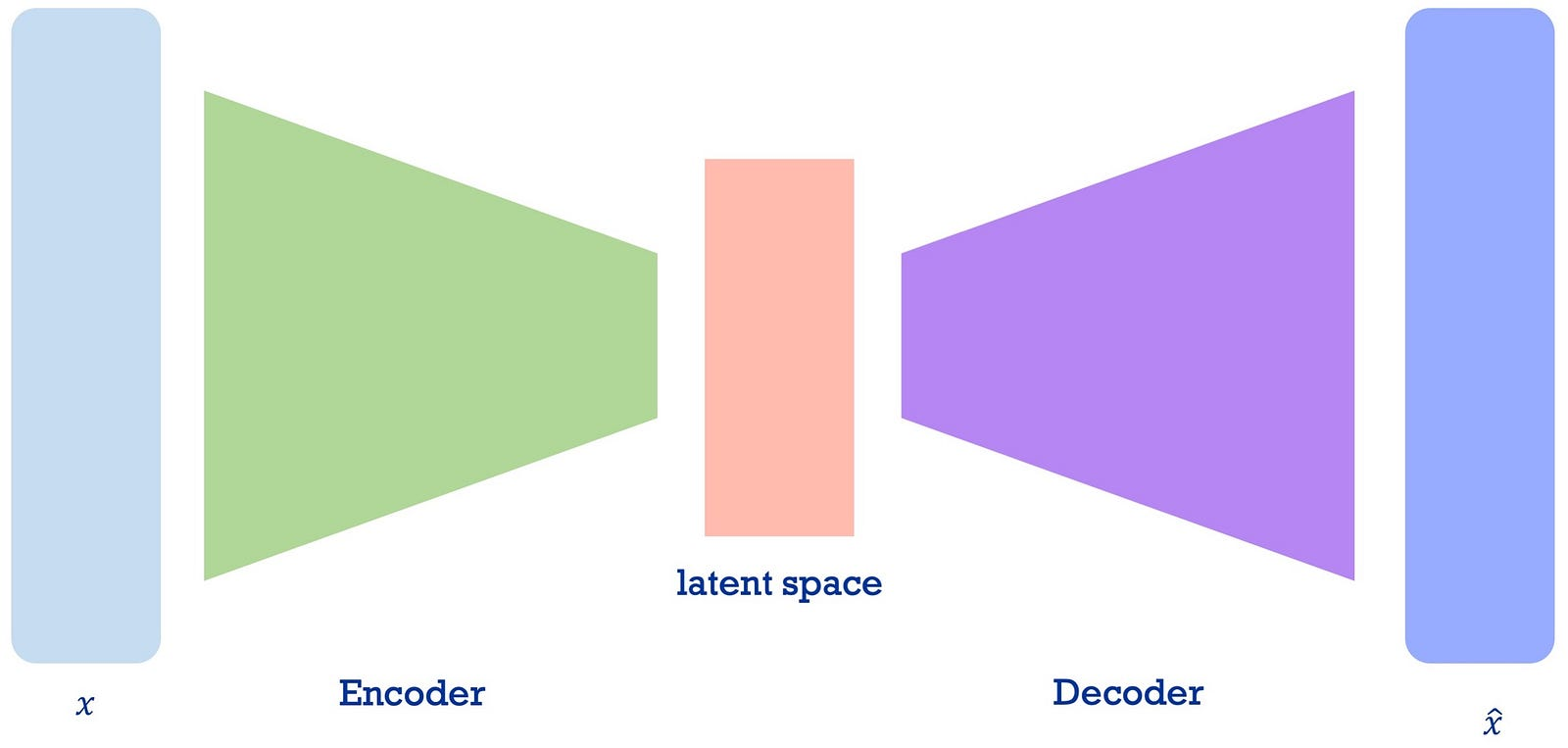Schematic representation of the AutoEncoder; after . On the left is the original domain space where true samples are drawn from; on the right is the reconstructed input. The visually smaller block in the middle represents the bottleneck layer, used to enforce compression of the data.

The first part of the network is the Encoder part, which maps (encodes) the features into aforementioned low-dimensional latent space. This encoding happens automatically, hence called AutoEncoder. From this encoded representation, the Decoder part tries to reconstruct the original data. This is an unsupervised technique, since true sample x and generated sample x’ can be compared directly, no label information is required.

The size of the latent space z influences the output quality; a larger space yields more accurately reconstructed samples x’. However, the decoder has no true generative capabilities (e.g., it can only re-construct samples, not invent them).

#### Technical aspect

The network is trained with the reconstruction loss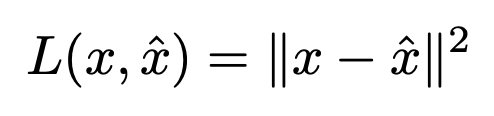Construction loss for the AutoEncoder, pairing the original and reconstructed sample for its similarity.

which is minimal when the distance between the input sample x and the reconstructed sample x’ is zero. In this case, the network has achieved a perfect reconstruction.

Think of a cat’s picture: You feed it to the model, and the more the resulting cat looks like your input, the better this model is. After all, you’d like to recognize your pet, would you not?

### Variational AutoEncoders

These generative capabilities can be enabled by adapting the latent space z, the idea behind Variational AutoEncoders (VAE) [2, 3, 4, 5; further resources: link 1, link 2].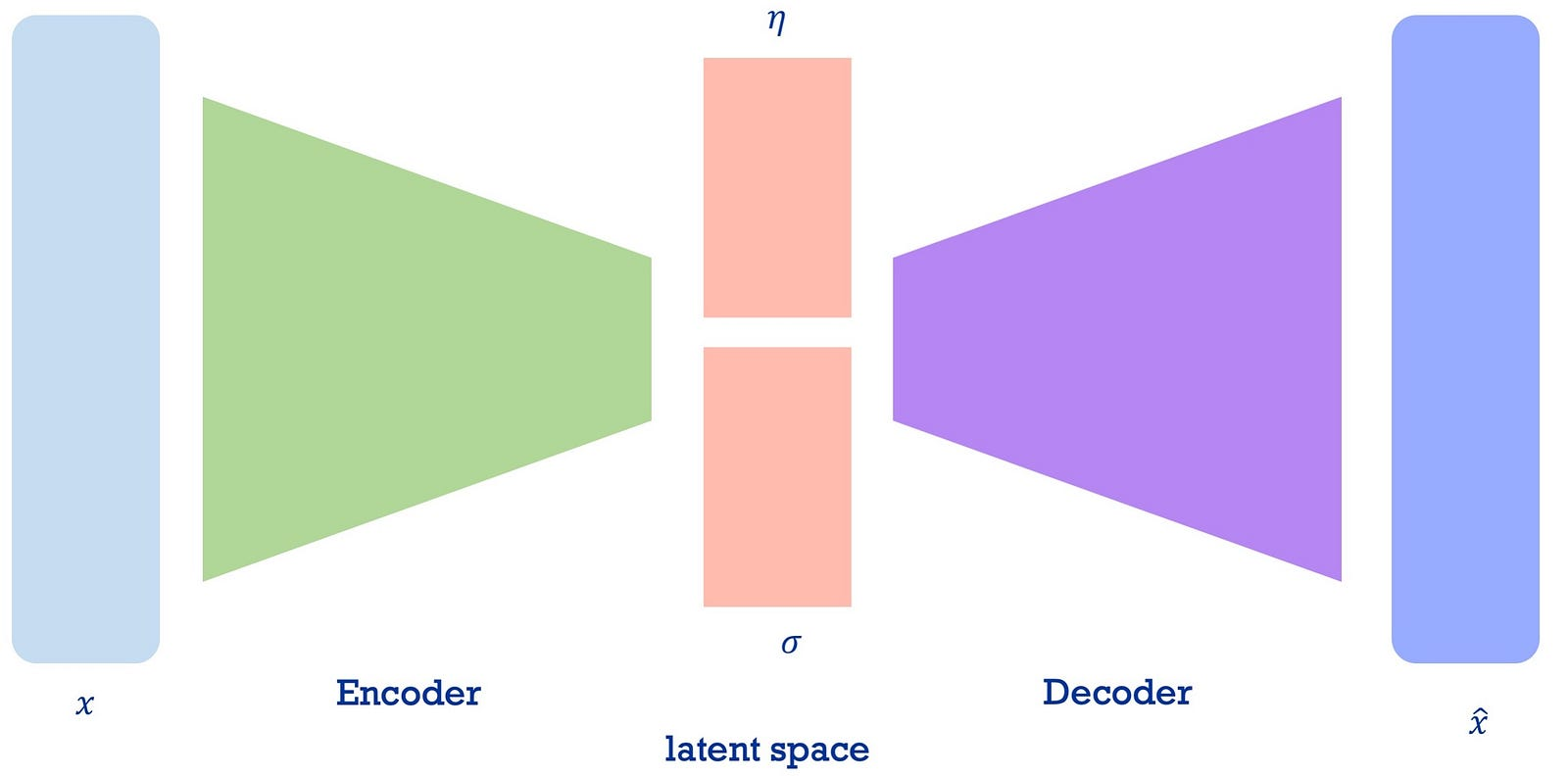Schematic representation of the Variational AutoEncoder, after . The key difference to the standard AutoEncoder setting is the modification of the latent space, which is split into a mean vector μ and standard deviation vector σ, which are the parameters of most probability distributions.

Similar to the AutoEncoder, it consists of an Encoder part and a Decoder part. The Encoder learns the mean μ and the standard deviation σ of the input data, it models a probability distribution for each dimension in the bottleneck layer. In the encoding process, a sample is drawn from the latent space. The output after the sampling layer is the sum of the mean vector μ and the deviation vector σ . Randomness (in other words, the creativity) is introduced by shifting μ and scaling σ with random constants η, drawn from a normal distribution. This (re-)parameterization trick separates the network and the probability parts, enabling backpropagation as usual .

Think of it this way:

You want to work with cat images (again). Instead of simply letting the network compress the picture, you have it learn the characteristics of the input samples. This could be the fur’s thickness, the tail’s length, the fur’s color, the ear’s size, and so on. That is why we have the μ and the σ as vectors: For each feature (fur, ears, tail, etc.), we model a probability distribution, learning how likely an attribute’s value (e.g. tail length) is. Generating a sample then mixes these attributes, to generate a new cat image — maybe of a yet-unknown race?

To prevent the network from memorizing its input samples, a regularization term is added to the loss function L, the Kullback-Leibler divergence. This divergence allows comparison between two probability distributions; in the VAE setting it is used to “distribute encodings [which would be our cat’s attributes] evenly around the center of the latent space” , and thus preventing memorization. Here, the latent space is understood as a probability distribution and compared to a prior distribution, an assumed distribution generating true samples x. This is commonly a unit Gaussian distribution, with mean 0 and variance 1.

The prior distribution captures our assumptions about how the data might be distributed. Usually, you want this to be as un-informative as possible, thus using a standard gaussian. For the cat’s setting, our distribution might say that the tail is not longer than 20 centimeters, or that the cat’s ears are usually between 5 and 10 centimetres of heigth.

#### Technical aspect

The basic VAE architecture utilizes two losses. The first one is the reconstruction loss — as in the AutoEncoder setting — , the second one is the regularization term, the mentioned Kullback-Leibler divergence. This divergence comes from the probability theory and is used in deriving parameters for a probability distribution (e.g. the mean or the variance). This fits the VAE setting, where these parameters have to be learned. A divergence function as used here is 0 if and only if two probability distributions are equal (in other words, when two random variables Y₁ and Y₂ are equal), and greater otherwise.

Adding the KL divergence to the loss enforces the latent space to come closer to a chosen prior. For the basic VAE, this prior is practically limited  to be the Gaussian distribution. Given both the network’s latent distribution and this prior, we have [2, 3]:

where Φ, Θ are the parameters (weights) of the encoder and decoder, R is the known reconstruction loss, and KL is the Kullback-Leibler divergence. This loss is minimal when the reconstructed sample does not differ from the input sample, and when the learned latent probability distribution is equal to the fixed prior.

Modeling artificial samples after a given dataset can be done directly by comparing the true data with the generated data, or indirectly by utilizing a downstream task that in turn enables the network to generate real samples. For direct approaches, one can use the Maximum Mean Discrepancy, which exceeds the scope of this post; see e.g.,  for further information.

The technique behind Generative Adversarial Networks (GANs)  relies on indirect comparison. In this framework, two networks are trained jointly: The Generator is trained to generate artificial samples from noise, looking as real as possible; and the Discriminator tries to distinguish them from real samples.

The noise input is a prior on the true data distribution, akin to the Gaussian prior in the VAE setting. The Generator learns a function that takes this simple distribution (the white noise) and transforms it into a complex distribution, representing the desired data. This transformative function is a complex function (meaning “not simple”), which neural networks have shown to learn exceedingly well; the networks weights are the function’s parameters. The Discriminator is the adversary of the Generator, a deep neural network mappings its input to a single scalar, outputting the probability that the input is a real sample.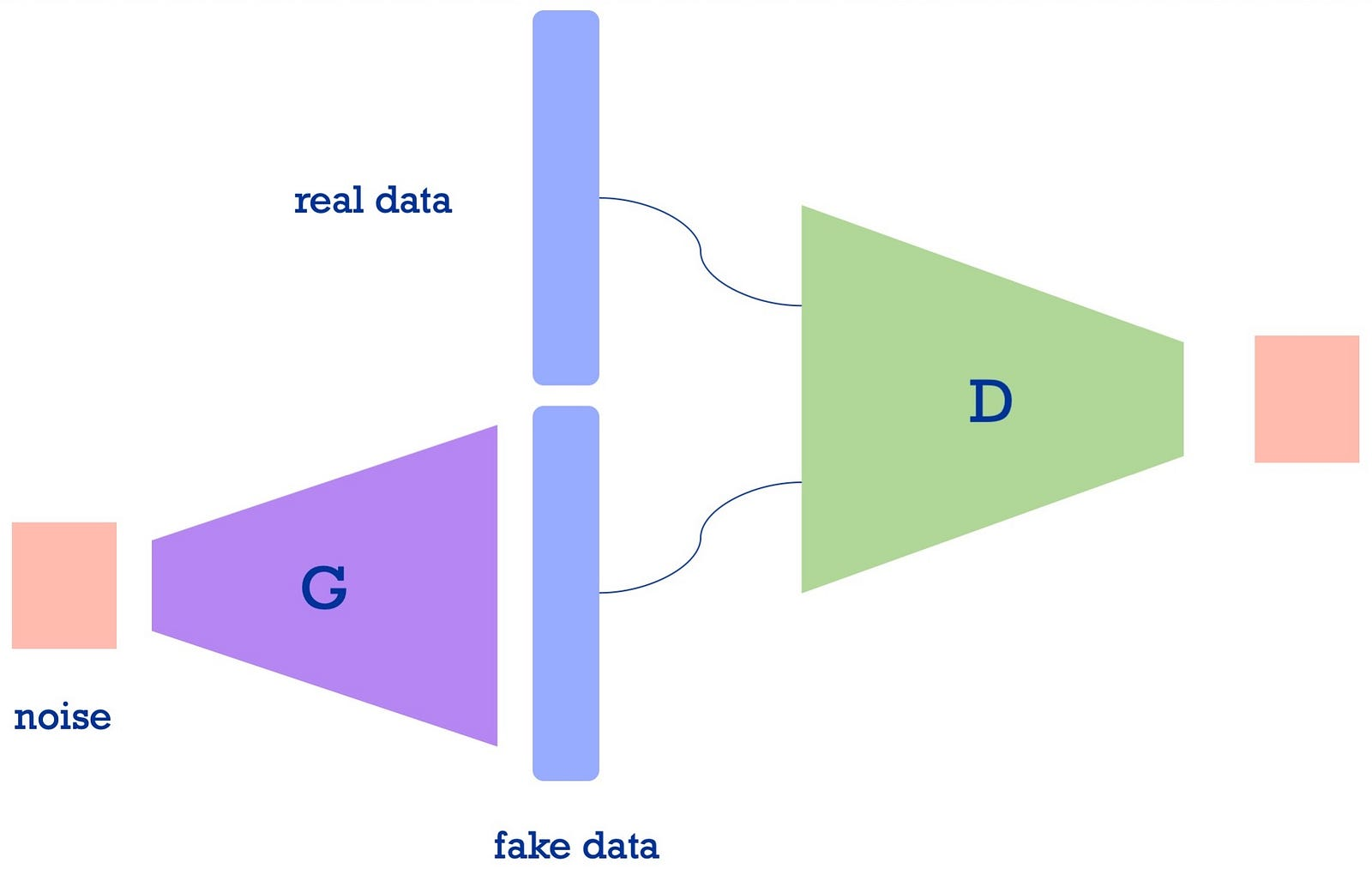Schematic representation of the GAN framework, after . On the left is the generator part learning the transformation from noise to the target domain, on the right is the discriminator part, distinguishing such generated fake samples from real data samples.

This process can be seen as two player min-max game. The equilibrium state in this game has the Generator generate indistinguishable fake samples, and the Discriminator always returning 0.5, i.e., guessing. The training procedure is described in algorithm 1 in : First, the Discriminator is updated for k steps, afterwards the Generator’s weights are updated. The last update happens by ascending the gradients; since the objective is to fool the Discriminator (i.e., increase the error), the ascent of the gradient has to be taken. Backpropagation updates the weights of the transformative function, which over time is nearing the desired distribution.

To have the Discriminator learn not only the distinction between real and fake samples, but also the distribution of the real data, it sees pairs of fake samples and real samples during training. This in turn enables the Generator to create more realistic looking samples.

For the cat-setting, we can collect various images of our pet, these are the true samples. Starting from noise, the Generator, guided by the Discriminator’s results, successively generates better samples. These cat photos might look blurry in the beginning, but over time they become more realistic. The Discriminator judges the quality (that is, the real-ness) of our generated images, by seeing true cats and generated cats.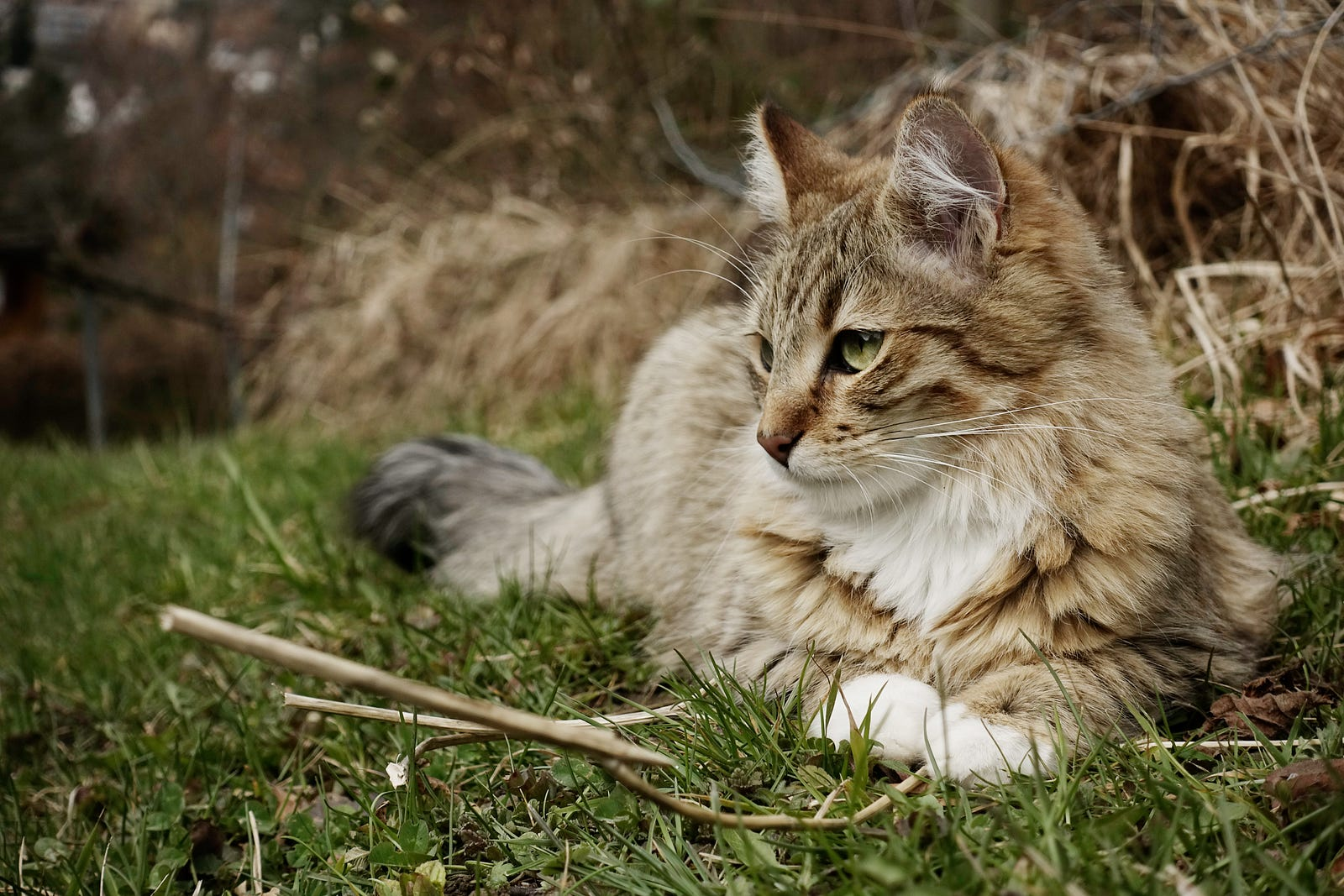A real cat, not a generated one! Photo by Sangia on Unsplash

#### Technical aspect

The idea is to generate a probability distribution that models the underlying distribution from the target domain. To achieve this, a prior p_z on the network input is defined , where z is a sample from that space. The generator network Gserves as the transformative function, turning the simple prior distribution into a complex distribution. Together with the discriminator, both follow

In this two player game, the generator tries to minimize the error it makes (hence min), and the discriminator is trained to maximize its classification accuracy (hence max).

### CycleGAN

An extension to the GAN framework is made with CycleGAN . This approach originally emerged to solve the problem of image to image translation, where the input image is from one domain (e.g., day), and the desired output is from another domain (e.g., night). Previous approaches required exhaustive and expensive datasets of paired images {mountain_day, mountain_night}, which is prohibitive for more complex domain changes. Secondly, generative processes are prone to a mode collapse, where all input samples are mapped to one single output sample ; this stales the training.

The CycleGAN approach solves this task on the set level; it takes unpaired sets of data from domain X and domain Y, no white noise input is required. The CycleGAN network aims to learn the underlying relation between these two domains, in both directions. The domain transfer x Xy Y is done by the generator G, whereas the transfer yx is done by F: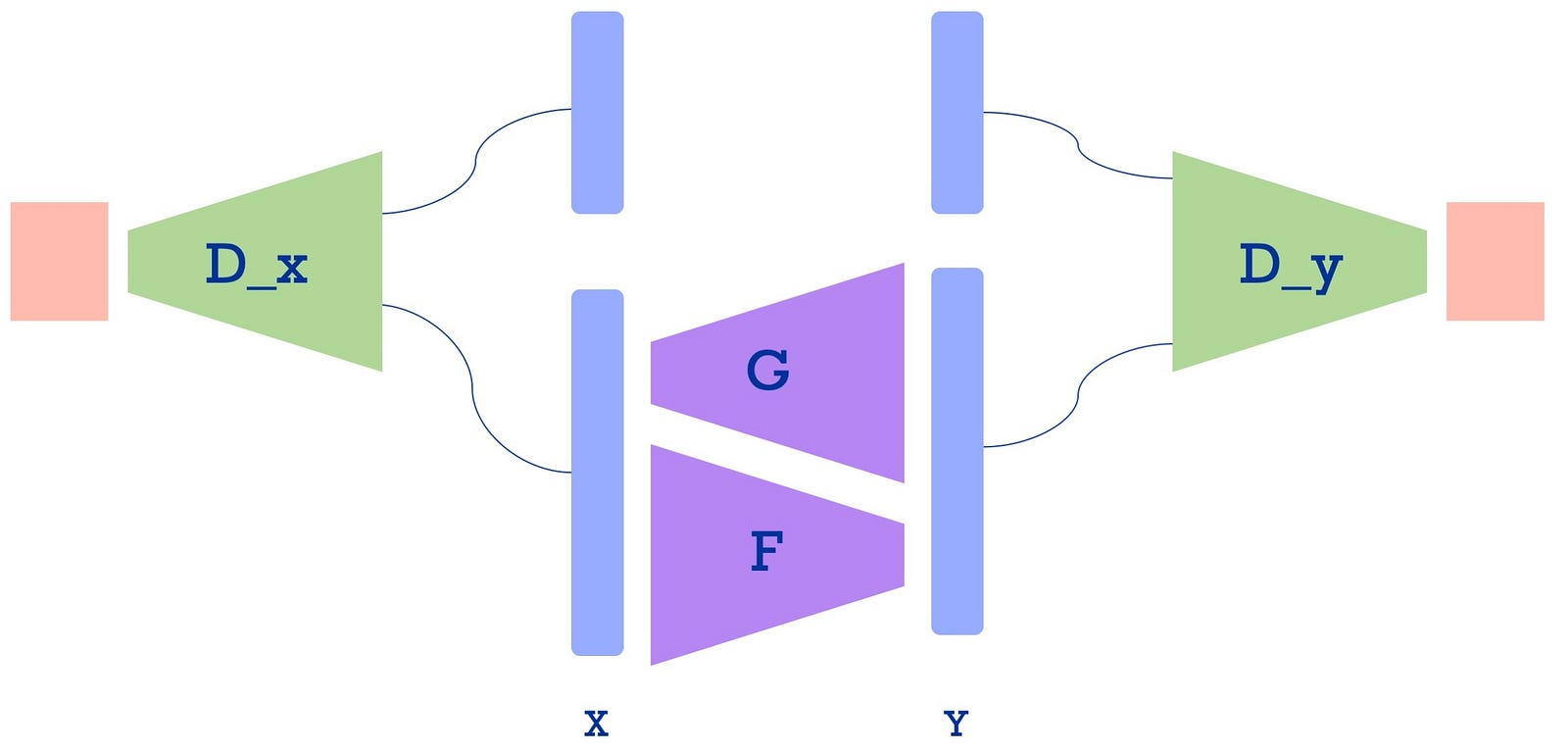Schematic representation of the CycleGAN framework; after . On the left discriminator D_x learns to distinguish between true samples of domain X and fake samples generated by F. On the right discriminator D_y learns to distinguish between real data of domain Y and fake samples generated by G.

Now, for our running cats example we might “convert” our pet to a tiger. We therefore collect images of cats and tigers. One generator converts our cat to a tiger, the other generator takes care of the backwards direction, creating a cat from a tiger.

Why do we even need this backwards direction?

To prevent the mode collapse: If we would have no implicit guarantee that the generated output is based on our input image, we could theoretically always return the same image of a cat-to-tiger mapping. Obviously, this would not be completely wrong, but it is not really what we are after.

The success of such a transfer is ensured by the cycle-consistency loss (which I think is something remarkably smart): Given an input x, a generator G generates y’. The second generator F transforms y’x’. Ideally, after the mapping to the Y domain and back — completing one cycle — , x’ is identical to x.

The practicality of a cyclic consistency can be seen in other domains as well: Translating an English sentence to French and back to English should yield the same original sentence .

The “quality” of the generated data (our cat images) is observed by a discriminator D_y, which distinguishes between true y samples and fake y samples. The quality of the second mapping is observed by D_x, which distinguishes between true samples x and generated samples. The total loss consists of the losses for both generator-discriminator  pairs and the cycle-consistency loss. The last loss prevents the aforementioned mode collapse by forcing each input to an exclusive output.

For our cats-tigers setup we have one discriminator which is an expert in classifying cats versus fake cats, and another expert discriminator for the tiger and fake tigers. The discriminators work against-with (if that’s even a word) the generators: The better the cat vs. fake cate discriminator gets, the better the tiger → cat generator get; similar for the other direction. This is why the GAN setting can be seen as a two player game.

#### Technical aspect

Extending the GAN setting, a CycleGAN can be seen as learning two complex transformative functions: from distribution A to B, and from B to A.

### Summary

The generative capabilities of deep neural networks have evolved over several years, with early methods using the AutoEncoder framework. Building on this, the Variational AutoEncoder adds stronger generative capabilities by randomly sampling from the latent space. A landmark was achieved when  proposed the Generative Adversarial Nets, enabling a model to learn the transformation from noise to a target domain. The CycleGAN framework extends this approach by using two generators and two discriminators to retain the original information contained in a sample.

In review, the gist is

• AutoEncoders compress and reconstruct their input
• Variational AutoEncoders add more generative techniques
• GANs (originally) use white noise as an input to generate data
• CycleGANs expand the GAN concept to make training more stable

That’s it for this post. For any corrections, comments, or remarks kindly leave a note. Thanks for reading!

#### Bibliography

In case you want to go beyond this overview, just consult the following resources:

 G. Hinton and R. Salakhutdinov, Reducing the dimensionality of data with neural networks (2006), Science

 A. Amini and A. Soleimany, Introduction to Deep Learning: deep generative models (2020), MIT lecture

 D. Kingma and M. Welling, Stochastic gradient VB and the variational auto-encoder (2014), Second International Conference on Learning Representations, ICLR

 D. Rezende et al., Stochastic Backpropagation and Approximate Inference in Deep Generative Models (2014), Proceedings of the 31st International Conference on Machine Learning (ICML)

 X. Chen et al., Variational Lossy Autoencoder (2017), arXiv

 A. Valenti et al., Learning Style-Aware Symbolic Music Representations by Adversarial Autoencoders (2020), 24th European Conference on Artificial Intelligence (ECAI2020)

 A. Gretton et al., A kernel method for the two-sample-problem (2007), Advances in neural information processing systems

 I. Goodfellow et al., Generative adversarial nets (2014), Advances in neural information processing systems

 J. Zhu et al., Unpaired image-to-image translation using cycle-consistent adversarial networks (2017), Proceedings of the IEEE international conference on computer vision

 Ian Goodfellow, NIPS 2016 tutorial: Generative adversarial networks (2016), arXiv